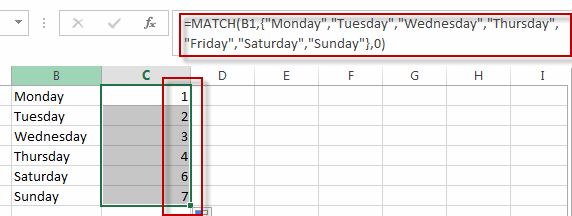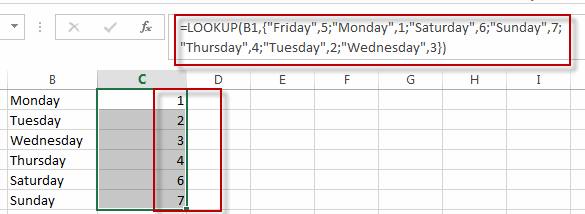# Convert Weekday Name to Number

This post will guide you how to convert weekday string to number in excel. How do I convert weekday name to number with a formula in excel. How to convert day of week to number in excel. Is there an easy way to convert a weekday string which is either “Monday” or “Tuesday” into a number in excel.

## Convert Weekday Name to Number

Assuming that you have list of data B1:B7 that contain weekday names, you want to convert all weekday names to numbers, how to achieve it.

You can use a formula based on the MATCH function to achieve the result. Just like this formula:

`=MATCH(B1,{"Monday","Tuesday","Wednesday","Thursday","Friday","Saturday","Sunday"},0)`

Type this formula into the formula box of one blank cell, such as: Cell C1, then press Enter key in your keyboard, and drag the AutoFill Handler over other cells to apply this formula to convert weekday string to numbers.You will see all weekday names in range B1:B7 have been converted to numbers in range C1:C7.

Or you can also use another complex formula based on the LOOKUP function to convert weekday names to numbers in excel. Like this:

`=LOOKUP(A1,{"Monday",1;"Friday",5;"Saturday",6;"Sunday",7;"Thursday",4;"Tues  day",2;"Wednesday",3})`### Related Functions

• Excel LOOKUP function
The Excel LOOKUP function will search a value in a vector or array.The syntax of the LOOKUP function is as below:= LOOKUP (lookup_value, lookup_vector, [result_vector])…
• Excel MATCH  function
The Excel MATCH function search a value in an array and returns the position of that item.The MATCH function is a build-in function in Microsoft Excel and it is categorized as a Lookup and Reference Function.The syntax of the MATCH function is as below:= MATCH  (lookup_value, lookup_array, [match_type])….

Related Posts

VLOOKUP From Another Sheet Not Working

In the previous post, you should know that how to fix or remove the #N/A error when using VLOOKUP formula to lookup value from another sheet. And this post will show you reasons why your VLOOKUP formula is not working ...

Case Sensitive Lookup with SUMPRODUCT and EXACT

Today, we will show you how to use SUMPRODUCT and EXACT to perform a case sensitive exact match. In this article, we provide a simple example to calculate bonus for employees whose names are case-sensitive. If you meet similar scenarios ...

Basic Usage of INDEX & MATCH – Case Sensitive Lookup

In Excel, INDEX function and MATCH function are often used together for retrieving data from a particular position. MATCH function is one of Excel lookup & reference functions that can perform approximate match or exact match by setting different match ...

Basic Rates Calculation by VLOOKUP Based on Weight Band

Microsoft Excel provides many functions that can execute logical test, search data, return current date and something else. They are very useful in daily work. And Excel VLOOKUP function is one of Excel most frequently used functions. It belongs to ...

Basic Grade Calculation by VLOOKUP Function – Approximate Match

In Excel, except combination INDEX+MATCH, we can also apply other functions to search data, for example VLOOKUP function. Like MATCH function, VLOOKUP function is one of Excel lookup & reference functions that can perform approximate match or exact match by ...

Basic Discount Calculation with VLOOKUP Function

In Excel, except combination INDEX+MATCH, we can also apply other functions to search data, for example VLOOKUP function. Like MATCH function, VLOOKUP function is one of Excel lookup & reference functions that can perform approximate match or exact match by ...

Basic Usage of INDEX & MATCH – Exact Match

In Excel, INDEX function and MATCH function are often used together for returning value or cell reference or range reference from specified position. And MATCH function is one of Excel lookup & reference functions that can perform approximate match or ...

Basic Usage of INDEX & MATCH – Approximate Match

In Excel, INDEX function and MATCH function are often used together for returning value or cell reference or range reference from specified position. And MATCH function is one of Excel lookup & reference functions that can perform approximate match by ...

Approximate Match with Multiple Criteria by INDEX & MATCH

In Excel, INDEX function and MATCH function are often used together for returning data from specific position. And MATCH function is one of Excel lookup & reference functions that can return approximate value by setting match type. Above all, through ...

How to Count Cells Not Equal to One of Many Things in Excel

This post will guide you how to count the number of cells that are not equal to many things in a given range cells using a formula in Excel 2013/2016. You can easily to count cells not equal to a ...

Sidebar